NCERT Exemplar Class 12 Maths Chapter 10 Vector Algebra are part of NCERT Exemplar Class 12 Maths. Here we have given Exemplar Problems for Class 12 Maths Chapter 10 Vector Algebra PDF.

## NCERT Exemplar Class 12 Maths Chapter 10 Vector Algebra

NCERT Exemplar Class 12 Maths Chapter 10 Vector Algebra Solutions is given below.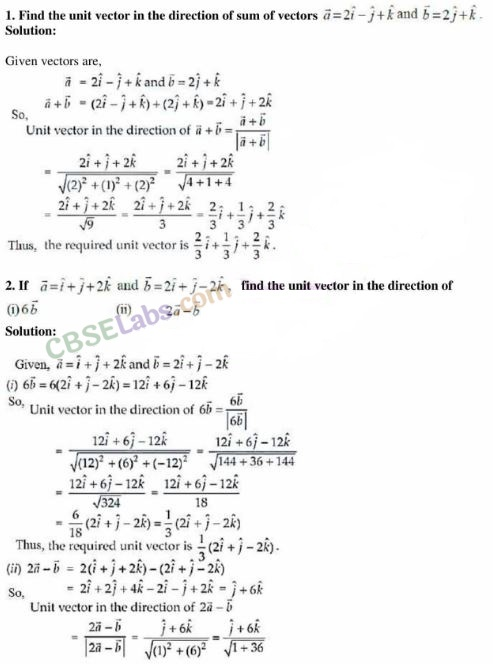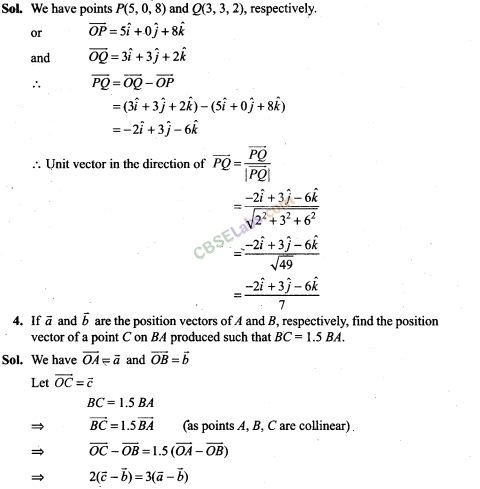5. Using vectors, find the value of k such that the points (k, -10, 3), (1, -1, 3) and (3, 5, 3) are collinear.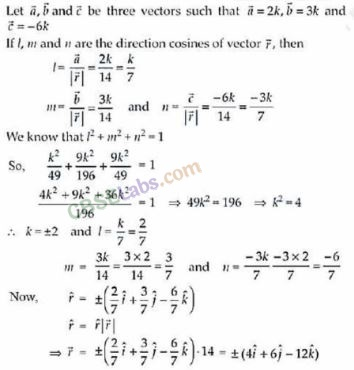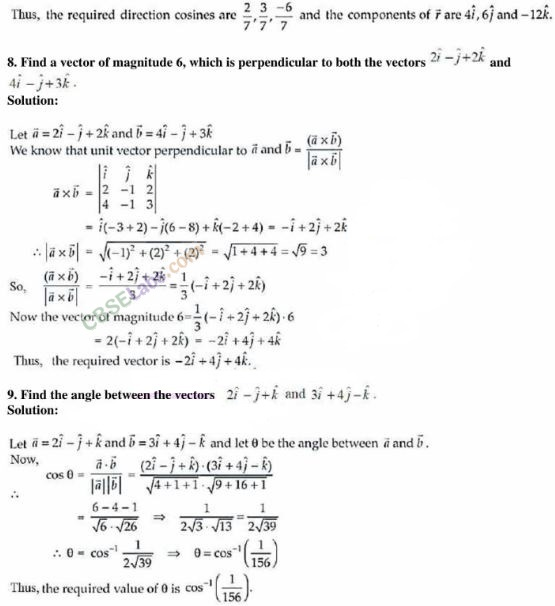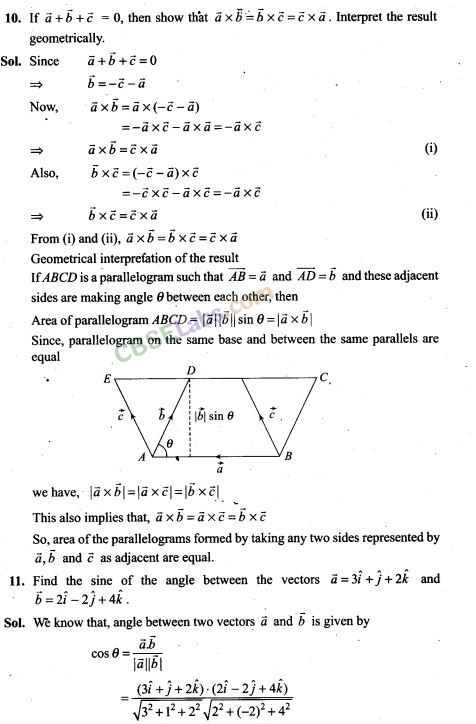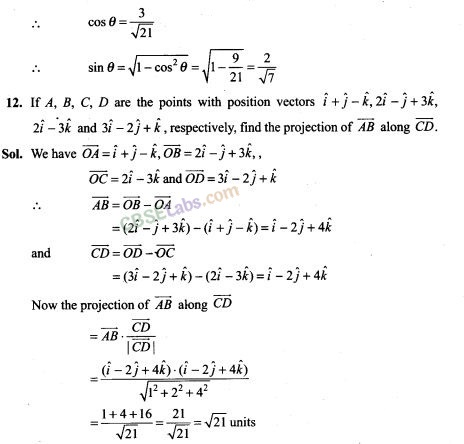13. Using vectors, find the area of the triangle ABC with vertices A(l, 2, 3), 5(2, -1, 4) and C(4,5,-l).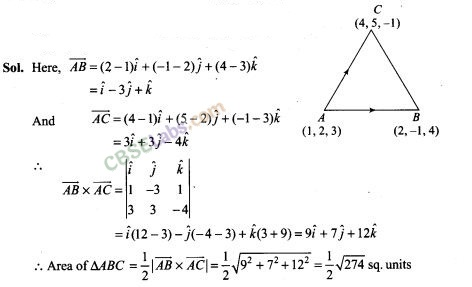14. Using vectors, prove that the parallelogram on the same base and between the same parallels are equal in area.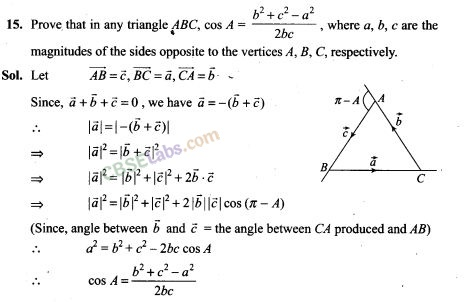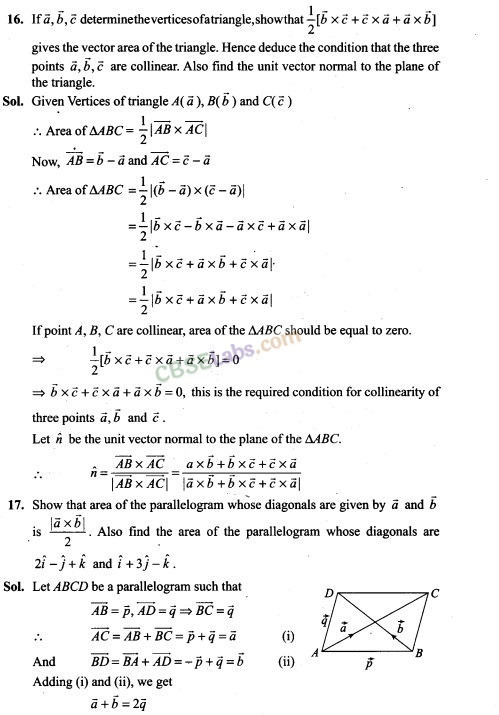Objective Type Questions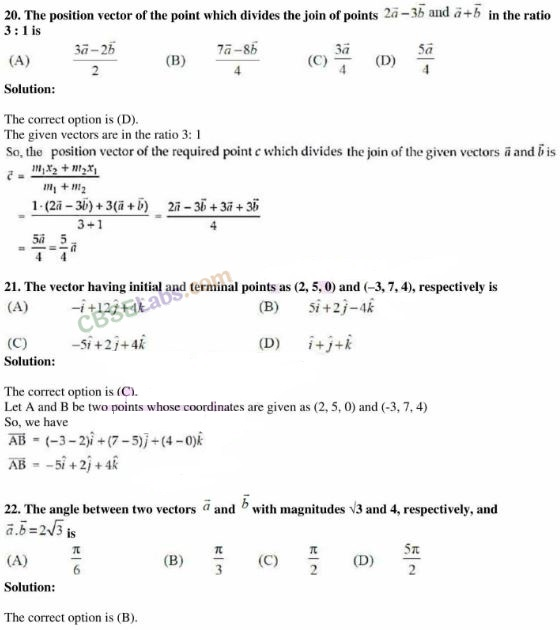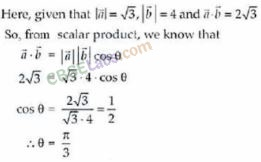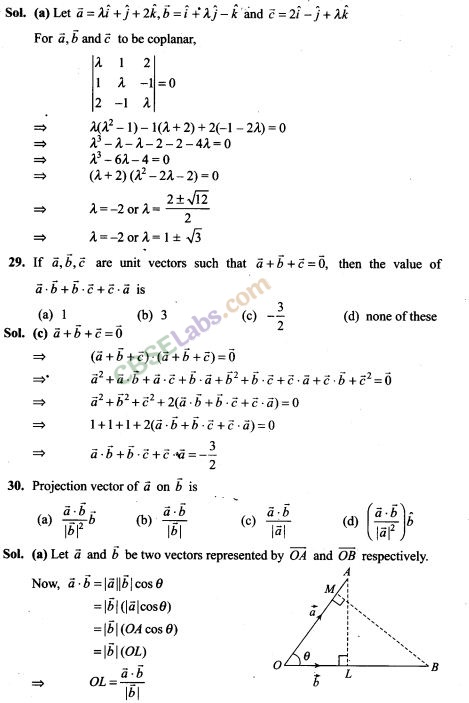Fill in the Blanks Type Questions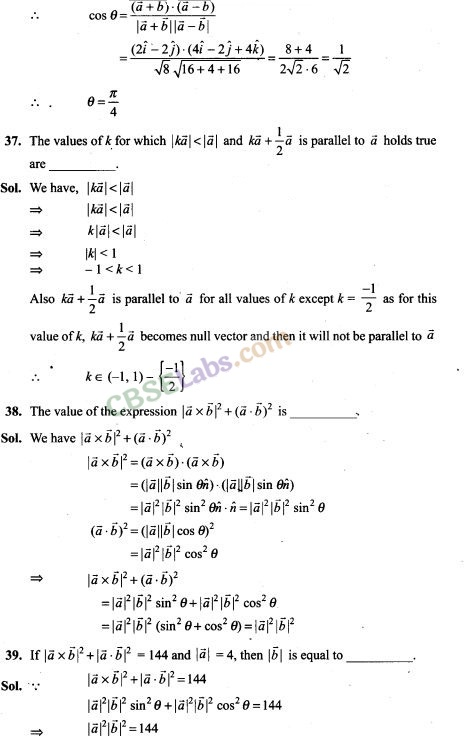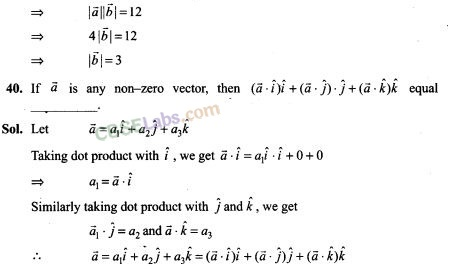True/False Type Questions

42. Position vector of a point P is a vector whose initial point is origin.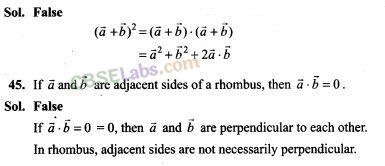## NCERT Exemplar Class 12 Maths Solutions

More Resources for Class 12 Maths

We hope the NCERT Exemplar Class 12 Maths Chapter 10 Vector Algebra help you. If you have any query regarding NCERT Exemplar Class 12 Maths Chapter 10 Vector Algebra, drop a comment below and we will get back to you at the earliest.